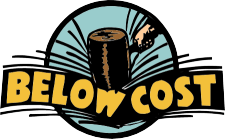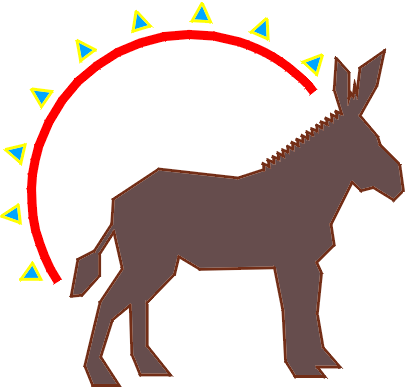The amount of increase or decrease in a value is the Percent of Increase or Decrease.

 Example: The computer was regularly priced at \$1500. If you order the computer over the internet, you can save money as the price is only \$1200. What percent discount are you getting? The regular price, which we will refer to as the "old" price is \$1500. The sales price, which we can call the "new" price is \$1200. The change in price or discount is \$1500-\$1200 = \$300.If we divide the discount by the "old" price, we arrive at the percent change… \$300÷\$1500 = .2 = 20% decreaseSlaphappy's Furniture Store is selling all their chairs at 7% below cost. Mark down the following prices and submit them to the manager, Ms. Wam.

 current price of chair reduced price \$46 \$ \$78 \$

Round all answers to the nearest penny.

In the first example, the "old" was more than the "new" and there was a decrease, so we called the change a discount.

In the next problem, there is an increase in price and we call this a markup in price.

Example: In 1992, the democratic support in a local community was about 47,000. In 1996, the support rose to 56,000. What was the percent increase?

 Old = 47000 New = 56000 Change or Markup = 56000- 47000 = 9000 Percent increase = 9000 ÷ 47000 = .1915 = 19.15%Using Formulas to Solve Percent Problems

 writer:Kevin Gallagher

Percent